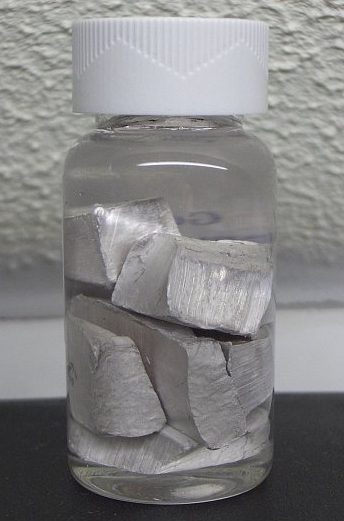Chemistry µGCSE:

Moles + Gases

10 quick questions - for GCSE and iGCSE

10 minutes maximum!

Grab a calculator and Periodic Table - time for some maths!

1. Which of these is the correct formula for the number of moles of a gas?
• A.  number of moles = gas volume (in cm3) x 24,000
• B.  number of moles = gas volume (in cm3) x 24
• C.  number of moles = gas volume (in cm3) ÷ 24,000
• D.  number of moles = gas volume (in cm3) ÷ 24
2. How many moles are there in 120 cm3 of fluorine gas, F2, at rtp?
[ Molar volume at rtp = 24,000 cm3]
• A.  200
• B.  5
• C.  0.005
• D.  3.163. What is the volume of 4 moles of oxygen gas at rtp?
[ Molar volume at rtp = 24 dm3]
• A.  96,000 dm3
• B.  6000 cm3
• C.  6 dm3
• D.  96 dm3
4. What volume does 1.6g of methane gas, CH4 , occupy at rtp?
[Mr of CH4 is 16;  Molar volume at rtp = 24,000 cm3]
• A.  2,400 cm3
• B.  2,400 dm3
• C.  38,400 cm3
• D.  15,000 cm3
5. In an experiment, 240cm3 of CO2 was released, what was the total loss in mass of the flask?
[Mr of CO2 is 44;  Molar volume at rtp = 24,000cm3]
• A.  440g
• B.  4.4g
• C.  0.44g
• D.  5.45g
6. 0.02 moles of sodium metal reacted vigorously with water in the following reaction:

2Na(s) + 2H2O(l)  2NaOH(aq) + H2(g)

What volume of hydrogen was produced, at rtp?

[Molar volume = 24,000cm3 at rtp]• A.   240cm3
• B.   480cm3
• C.   960cm3
• D.   10cm3

7. 50cm3 of nitrogen monoxide reacted with oxygen. The equation for this reaction is:

2NO (g) + O2 (g) 2NO2 (g)

How many moles of nitrogen monoxide were used and what volume of oxygen was required?
[Molar volume = 24,000cm3 at rtp]

 Moles of nitrogen monoxide used Volume of oxygen required/cm3 A 0.0021 50 B 0.0021 25 C 0.0042 50 D 0.0042 25

Q8+9.
Carbon monoxide gas was used to reduce 320kg of iron(III)oxide to iron.
The equation for the reaction is:

Fe2O3(s)  + 3 CO(g)  2 Fe(s) + 3 CO2(g)

8. How many moles of Fe2O3 were reduced?
[Ar of Fe = 56; Ar of O = 16]
• A.   2.0
• B.   4.4
• C.   2000
• D.   4400
9. What volume of carbon monoxide, at rtp, would be needed for this reduction?
[Molar volume = 24dm3 at rtp]
• A.   144,000 dm3
• B.   48,000 dm3
• C.   144 dm3
• D.   48 dm3

10. During an exothermic reaction, calcium carbonate reacted with excess acid producing 0.005 moles of carbon dioxide gas.

If 140cm3 of carbon dioxide was collected (not at rtp), what was the molar volume of gas under these conditions?

• A.  120 cm3
• B.  24,000 cm3
• C.  120 dm3
• D.  28,000 cm3